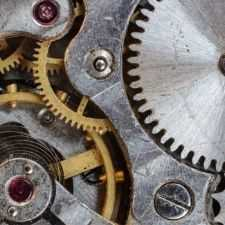Mechanical
Community of Mechanical Engineers: Get help about everything related to mechanical engineering from fellow mechanical engineers.
805 Members
Join this group to post and comment.zaveri • Mar 27, 2012

# mechanical power

what is the formula to calculate the output mechanical power for the following prime movers ?

1) electric motor
2) I.C engine
3) hydraulic turbine (pelton , kaplan, francis)
4) hydraulic rotary actuator

also the input power for an electric motor is the product of voltage and current.
(P = V * I)

similarly what is the formula to calculate the power inputed to the rest of the machines ?